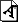# Supersonic Flow Simulation on Emulated Digital Cellular Neural Networks

A wide range of industrial processes and scientific phenomena involve gas or fluids flowing over complex obstacles, eg the flow of air around vehicles or buildings, or that of water in the oceans. In engineering applications the temporal evolution of non-ideal, compressible fluids is often modelled by the system of Navier-Stokes equations. By neglecting all non-ideal processes and assuming adiabatic variations, we obtain the Euler equations, describing the dynamics of dissipation-free, inviscid, compressible fluids. They are a coupled set of nonlinear hyperbolic partial differential equations and form a relatively simple yet efficient model of compressible fluid dynamics. Unfortunately, the necessity of the coupled multi-layered computational structure with nonlinear, space-variant templates does not make it possible to utilize the huge computing power of the analogue Cellular Neural Network Universal Machine (CNN-UM) chips. To improve the performance of our solution, emulated digital CNN-UM implemented on field programmable gate arrays (FPGAs) has been used. Thus, we intend to perform the operations with the highest possible parallelism.

Since the logically structured arrangement of data is fundamental for the efficient operation of the FPGA-based implementations, we consider explicit second-order accurate finite-volume discretization of the governing equations over structured mesh employing a simple numerical flux function. The main advantage of this method over the forward Euler method, which is used extensively in the computation of the CNN dynamics, is that this approximation is more robust in the case of complex computational geometries and in the presence of shock waves in the solutions. Indeed, the corresponding rectangular arrangement of information and the choice of a multi-level temporal integration strategy ensure the continuous flow of data through the CNN-UM architecture.

The Falcon architecture is an emulated digital implementation of CNN-UM array processor that uses the full signal range model. On this architecture the flexibility of simulators is combined with the computational power of analogue devices. Not only can the template size and computational precision be configured, but space-variant and nonlinear templates can also be used. In accordance with the discretized governing equations, we have designed a complex circuit that is able to update the values of the conservative state vector of a cell in every clock cycle using the emulated digital CNN-UM architecture.

Implementation and testing of such an application-specific arithmetic unit can be very time-consuming. However, using rapid prototyping techniques and high-level hardware description languages such as Handel-C from Agility makes it possible to develop the optimized arithmetic unit much faster than using an approach based on conventional hardware description language (HDL).Figure 1: The density values of the Mach 3 flow after 0.4s, 1s and 4s computed in a model having an array size of 320×960.

To show the efficiency of our solution, we used a complex test case in which the flow profile over a forward-facing step was computed. The simulated region is a two-dimensional cut of a pipe that is closed at the upper and lower boundaries and open at the left and right boundaries. The direction of flow is from left to right and the flow speed at the left boundary is constant and set at three times the speed of sound (Mach 3). The solution contains shock waves reflecting from the closed boundaries. Figure 1 shows the results of the computation using the derived method after 0.4s, 1s and 4s of simulation time with a 0.39ms (1/2560s) time step.

The proposed circuit can be implemented on mid-sized gate arrays on Agility RC203 and RC2000 boards. In the case of second-order approximation, an approximately 21-fold speedup can be achieved compared to the Intel Core2Duo T7200 processor running at 2 GHz. By using larger FPGAs, the achievable performance can be improved more than 589-fold.

In future the designed arithmetic unit will be extended to three-dimensional flow problems and non-uniform computational Grids could be possible.

E-mail: skocsovision.vein.hu